## PAFEC VibroAcoustics - Large displacement analysis of a spider

 The spider (suspension / outer centering device (OCD)) is excited via the former. At low frequencies the former tends to vibrate with relatively large amplitude. It is important to avoid distortion of the spider due to non-linearity within the normal operating range, but beneficial if the stiffness increases for larger extensions, to prevent "jump out" from the gap. With the corrugated design, small axial movement is opposed by the bending stiffness. Near maximum displacement as the corrugations begin to flatten out, membrane stiffness (material) properties predominate. Finite element analysis can be used to compute the load/displacement curve.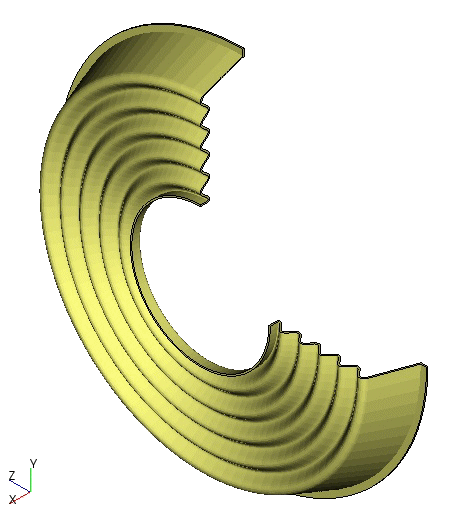270o cut-away of spider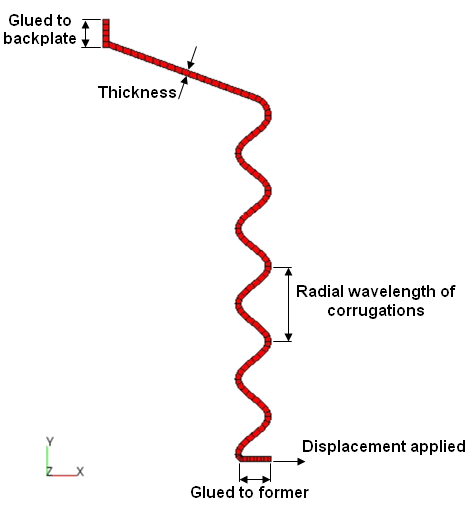Finite element model of a loudspeaker spider Axisymmetric generator plane modelled.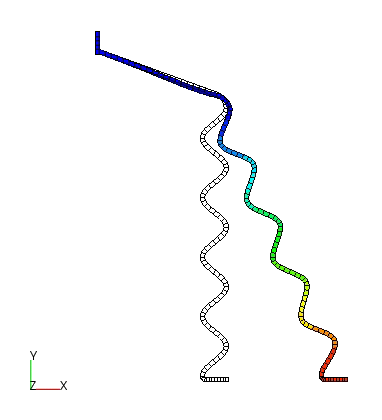Maximum positive deformation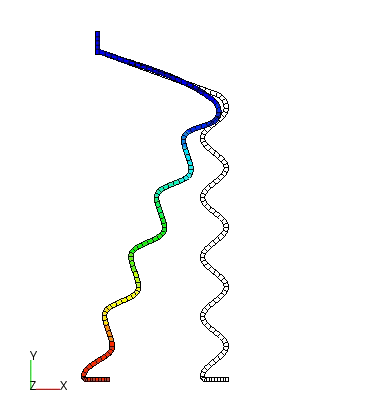Maximum negative deformation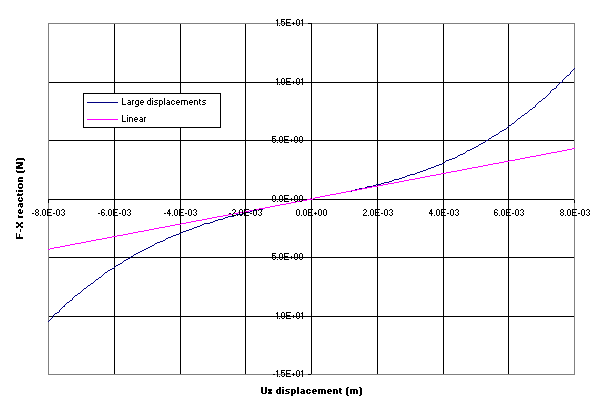At small displacements the strains and rotations are assumed to be infinitesimal and the load displacement response is linear; as can be seen by the central linear section of the large displacement graph (blue line). When the deformation includes large rotations, the assumptions of linear theory are no longer appropriate, and it becomes necessary to choose a more accurate strain measures (objective). The large displacement solution shown opposite is based on a total Lagrangian formulation using the logarithmic strain.